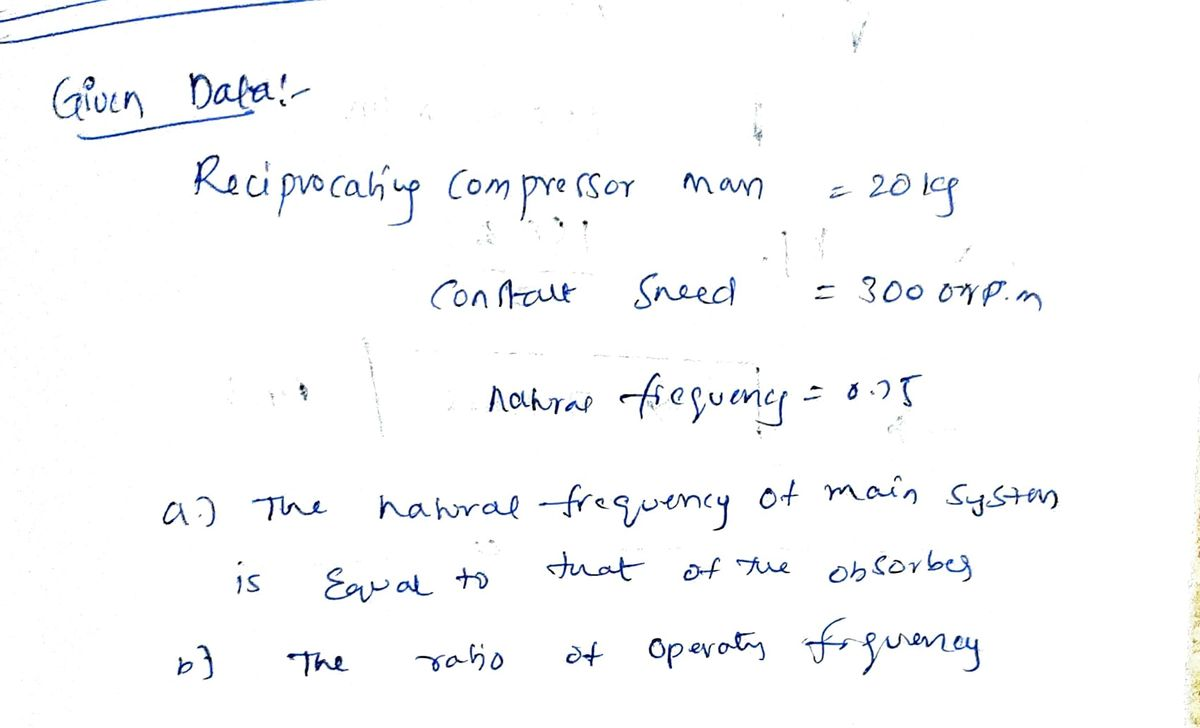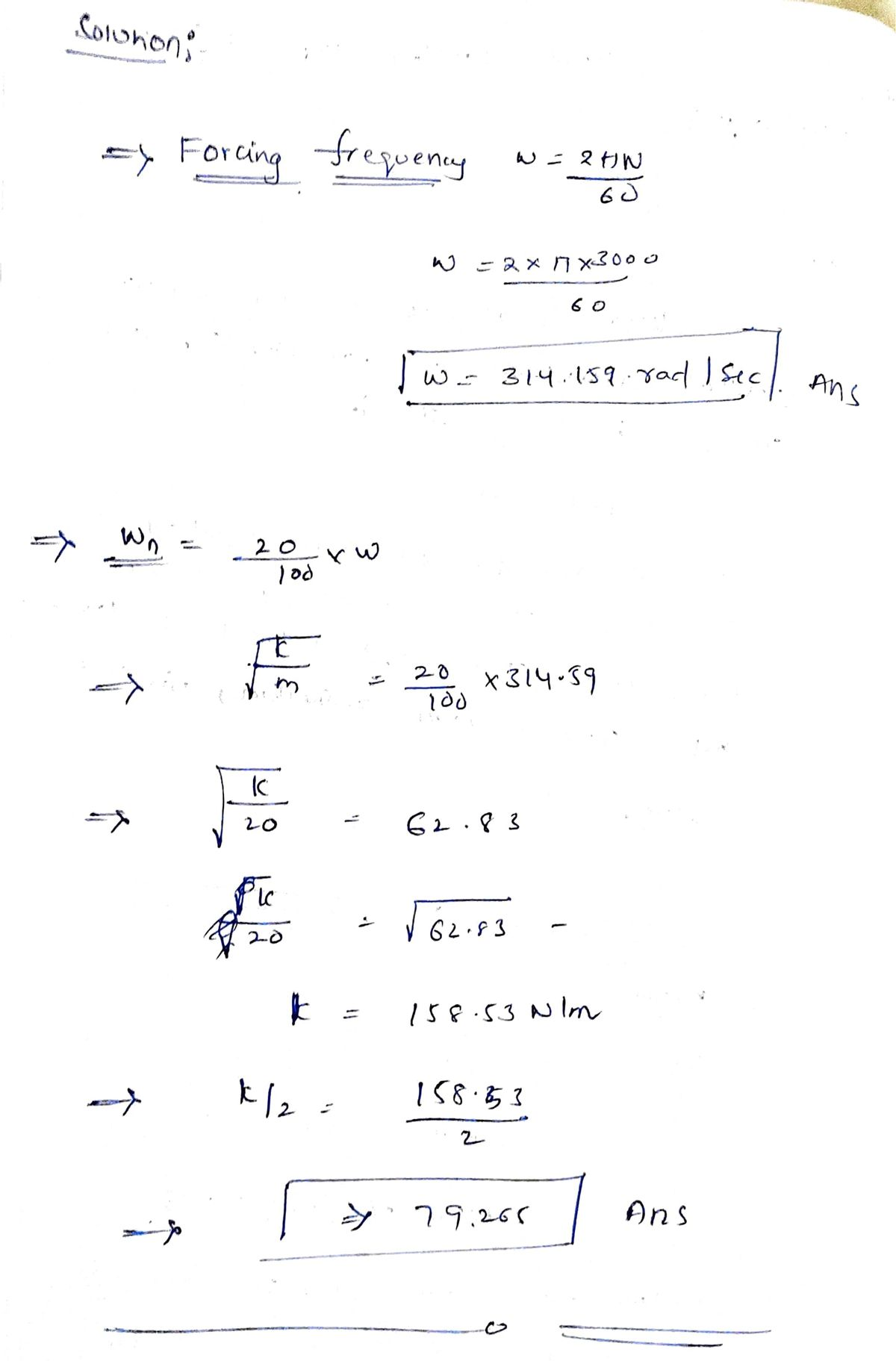# A reciprocating compressor of mass 20 Kg runs at a constant speed of 3000 rpm. After installation the forcing frequency is found to be too closer to the natural frequency of the system. Design a dynamic vibration absorber such that (a) the natural frequency of the main system is equal to that of the absorber and (b) the ratio of the operating frequency to the natural frequency is 0.75.

Question-AnswerCategory: Mechanical EngineeringA reciprocating compressor of mass 20 Kg runs at a constant speed of 3000 rpm. After installation the forcing frequency is found to be too closer to the natural frequency of the system. Design a dynamic vibration absorber such that (a) the natural frequency of the main system is equal to that of the absorber and (b) the ratio of the operating frequency to the natural frequency is 0.75.

A reciprocating compressor of mass 20 Kg runs at a constant speed
of 3000 rpm. After installation the forcing frequency is found to be too
closer to the natural frequency of the system. Design a dynamic
vibration absorber such that (a) the natural frequency of the main
system is equal to that of the absorber and (b) the ratio of the
operating frequency to the natural frequency is 0.75.